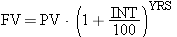Complete the program below that prompts for an interest rate (INT) and prints out a table of the future values (FV) of a certificate of deposit (CD) that begins at \$1,000.00 (present value or PV) for different numbers of years (YRS): 0, 5, 10, 15, 20, 25. You should use the following formula for future value (FV):Use the Math.pow() method shown in section 2.5.3.

While the number of years may be an integer, all amounts of money and all interest rates should be double variables. The future value amounts (FV) should be printed out to two decimal places.

For the interest rate of 7.50%, the table should look like this:

``` 0 1000.00
5 1435.63
10 2061.03
15 2958.88
20 4247.85
25 6098.34
```

Complete the following file: